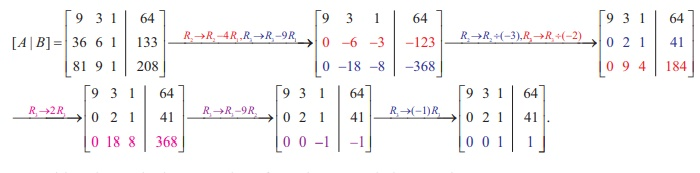Home | | Maths 12th Std | Solved Example Problems on Applications of Matrices: Solving System of Linear Equations

# Solved Example Problems on Applications of Matrices: Solving System of Linear Equations

Solution to a System of Linear equations: (i) Matrix Inversion Method (ii) CramerÔÇÖs Rule (iii) Gaussian Elimination Method

Solution to a System of Linear equations

## (i) Matrix Inversion Method

### Example 1.22

Solve the following system of linear equations, using matrix inversion method:

5+ 2 = 3, 3+ 2 = 5 .

### Solution

The matrix form of the system is AX = B , whereWe find |A| == 10 - 6= 4 Ôëá 0. So, AÔłĺ1  exists and AÔłĺ1 =Then, applying the formula X = AÔłĺ1B , we getSo the solution is (x = Ôłĺ1, y = 4).

### Example 1.23

Solve the following system of equations, using matrix inversion method:

2x1 + 3x2 + 3x3 = 5,

x1 ÔÇô 2x2 + x3 = -4,

3x1 ÔÇô x2 ÔÇô 2x3 = 3

### Solution

The matrix form of the system is AX = B,whereSo, the solution is ( x1 = 1, x2 = 2, x3 = Ôłĺ1) .

Example 1.24

If, find the products AB and BA and hence solve the system of equations x Ôłĺ y + z = 4, x ÔÇô 2y ÔÇô 2z = 9, 2x + y +3z =1.

SolutionWriting the given system of equations in matrix form, we getHence, the solution is (x = 3, y = - 2,  z = Ôłĺ1).

## (ii) CramerÔÇÖs Rule

### Example 1.25

Solve, by CramerÔÇÖs rule, the system of equations

x1 Ôłĺ x2 = 3, 2x1 + 3x2 + 4x3 = 17, x2 + 2x3 = 7.

### Solution

First we evaluate the determinantsSo, the solution is (x1 = 2, x2 = - 1,  x3 = 4).

### Example 1.26

In a T20 match, Chennai Super Kings needed just 6 runs to win with 1 ball left to go in the last over. The last ball was bowled and the batsman at the crease hit it high up. The ball traversed along a path in a vertical plane and the equation of the path is = ax2 + bx + with respect to a xy -coordinate system in the  vertical  plane  and  the  ball  traversed   through   the   points  (10,8), (20,16), (30,18) , can you conclude that Chennai Super Kings won the match?Justify your answer. (All distances are measured in metres and the meeting point of the plane of the path with the farthest boundary line is (70, 0).)

### Solution

The path  axbx c  passes through the points (10,8), (20,16), (40, 22) . So, we get the system of equations 100a + 10b + c = 8, 400a + 20b + c= 16,1600a + 40b + c = 22. To apply CramerÔÇÖs rule, we findWhen = 70, we get = 6.

So, the ball went by 6 metres high over the boundary line and it is impossible for a fielder standing even just before theboundary line to jump and catch the ball.

Hence the ball went for a super six and the Chennai Super Kings won the match.

## (iii) Gaussian Elimination Method

### Example 1.27

Solve the following system of linear equations, by Gaussian elimination method :

4+ 3+ 6= 25, + 5 + 7= 13, 2+ 9 + = 1.

### Solution

Transforming the augmented matrix to echelon form, we getThe equivalent system is written by using the echelon form:

x + 5y  + 7z = 13 , ÔÇŽ (1)

17y + 22z = 27 , ÔÇŽ (2)

199z = 398 . ÔÇŽ (3)Substituting z = 2, y = -1  in (1), we get x = 13 - 5  ├Ś (Ôłĺ1 ) Ôłĺ 7 ├Ś 2 = 4 .

So, the solution is ( x =4, y = - 1, z = 2 ).

Note. The above method of going from the last equation to the first equation is called the method of back substitution.

### Example 1.28

The  upward  speed  v(t) of a rocket  at time t is approximated by v(t) = at2 + bt + c, 0 ÔëĄ  t ÔëĄ 100 where a, b, and c are constants. It has been found that the speed at times t = 3, t = 6 , and t = 9 seconds are respectively, 64, 133, and 208 miles per second respectively. Find the speed at time  t = 15 seconds. (Use Gaussian elimination method.)### Solution

Since v(3) =64, v(6) = 133 and v(9) = 208 , we get the following system of linear equations

9a +3b + c = 64 ,

36a + 6b + c = 133,

81a + 9b + c = 208 .

We solve the above system of linear equations by Gaussian elimination method.

Reducing the augmented matrix to an equivalent row-echelon form by using elementary row  operations, we getWriting the equivalent equations from the row-echelon matrix, we get

9a + 3b + c =  64, 2b + c = 41, c= 1.

By back substitution, we getSo, we get v (t) = 1/3 t2  + 20t + 1.

Hence, v(15) = 1/3 (225) + 20(15) + 1 = 75 + 300 + 1 = 376

Tags : Definition, Formulas, Solved Example Problems , 12th Mathematics : UNIT 1 : Applications of Matrices and Determinants
Study Material, Lecturing Notes, Assignment, Reference, Wiki description explanation, brief detail
12th Mathematics : UNIT 1 : Applications of Matrices and Determinants : Solved Example Problems on Applications of Matrices: Solving System of Linear Equations | Definition, Formulas, Solved Example Problems